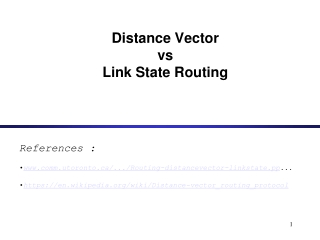DownloadDownload PresentationDistance Vector vs Link State Routing

# Distance Vector vs Link State Routing

Download Presentation## Distance Vector vs Link State Routing

- - - - - - - - - - - - - - - - - - - - - - - - - - - E N D - - - - - - - - - - - - - - - - - - - - - - - - - - -
##### Presentation Transcript

1. Distance Vector vs Link State Routing References : • www.comm.utoronto.ca/.../Routing-distancevector-linkstate.pp... • https://en.wikipedia.org/wiki/Distance-vector_routing_protocol

2. Routing • Recall: There are two parts to routing IP packets: 1. How to pass a packet from an input interface to the output interface of a router (packet forwarding) ? 2. How to find and setup a route ? • Packet forwarding is done differently in datagram and virtual-circuit packet networks • Route calculation is done in a similar fashion

3. Routing Algorithms • Objective of routing algorithms is to calculate `good’ routes • Routing algorithms for both datagrams and virtual circuits should satisfy: - Correctness - Simplicity - Simplicity - Robustness - Stability - Fairness - Optimality • Impossible to satisfy everything at the same time

4. Shortest-Path Routing • Routing algorithms generally use a shortest path algorithm to calculate the route with the least cost • Three components: 1. Measurement Component • Nodes (routers) measure the current characteristics such as delay, throughput, and “cost” 2. Protocol • Nodes disseminate the measured information to other nodes 3. Calculation • Nodes run a least-cost routing algorithm to recalculate their routes

5. Approaches to Shortest Path Routing • There are two basic approaches to least-cost routing in a communication network • There are two basic approaches to shortest-path routing: 1. Link State Routing 2. Distance Vector Routing

6. Approaches to Shortest Path Routing • 1. Link State Routing • Each node knows the distance to its neighbors • The distance information (=link state) is broadcast to all nodes in the network • Each node calculates the routing tables independently 2. Distance Vector Routing • Each node knows the distance (=cost) to its directly connected neighbors • A node sends a list to its neighbors with the current distances to all nodes • If all nodes update their distances, the routing tables eventually converge

7. Distance Vector Routing

8. Distance Vector • Each node maintains two tables: • Distance Table: Cost to each node via each outgoing link • Routing Table: Minimum cost to each node and next hop node • Nodes exchange messages that contain information on the cost of a route • Reception of messages triggers recalculation of routing table

9. Distance Vector – Bellman Ford algorithm

10. Distance Vector – Bellman Ford algorithm

11. Distance Vector – Bellman Ford algorithm

12. Distance Vector – Bellman Ford algorithm

14. Distance Vector vs. Link State Routing • With distance vector routing, each node has information only about the next hop: • Node A: to reach F go to B • Node B: to reach F go to D • Node D: to reach F go to E • Node E: go directly to F • Distance vector routing makespoor routing decisions if directions are not completelycorrect (e.g., because a node is down). • If parts of the directions incorrect, the routing may be incorrect until the routing algorithms has re-converged. A B C F D E

15. A A A A A A B B B B B B C C C C C C F F F F F F D D D D D D E E E E E E Distance Vector vs. Link State Routing • In link state routing, each node has a complete map of the topology • If a node fails, each node can calculate the new route • Difficulty:All nodes need to have a consistent view of the network A B C F D E

16. Link State Routing • Each node must • discover its neighbors • measure the delay (=cost) to its neighbors • broadcast a packet with this information to all other nodes • compute the shortest paths to every other router • The broadcast can be accomplished by flooding • The shortest paths can be computer with Dijkstra’s algorithm